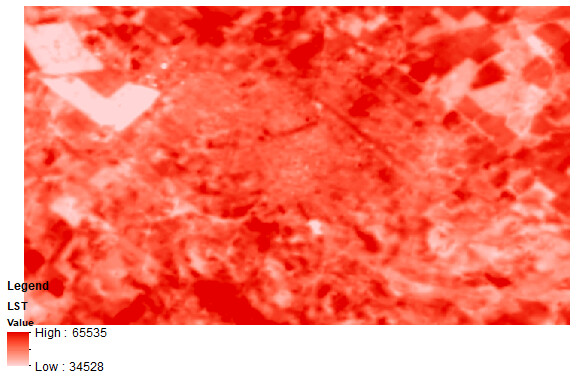# LST values using custom-script

Hi everyone!

I used the custom script to calculate the Land Surface Temperature (Land Surface Temperature (LST) Mapping Script | Sentinel-Hub custom scripts) from Landsat 8. I downloaded the image and then I used ArcMap to see the values. The values are strange, they’re not in Kelvin or Celsius.

I don’t know what I could be doing wrong or if it is necessary to do some other calculations after copying the script. Here is an example.

Could you help me? Thanks in advance!It is because the script is returning the visualisation instead of the LST values.

``````let viz = ColorGradientVisualizer.createRedTemperature(minC, maxC);
return viz.process(outLST)
``````

The above `ColorGradientVisualizer.createRedTemperature` is taken from here which visaulises LST as red gradient colors.

Best Regards

Thank you for your response. Does it mean that I have to change the return? What would be the correct code? Sorry for asking again!

Kind regards

Yes, you need to modify the code to obtain the result you’re interested in. This script could actually be a really good starting point. Here’s my suggested steps:

1. Figure out how LST is calculated in this script. The code used to calculate LST is `LSTi=(b10BTi/(1+(((bCent*b10BTi)/rho)*Math.log(LSEi))))`
2. The calculation in this script contains several constants, e.g., `NDVIs` for bare soil, `NDVIv` for full vegetation, `C` for surface roughness, etc. These constants can be area specific, so you may want to figure out the proper values for your area of interest.
3. Try to apply you calculation in the evalscript by modifying this example. The only difference is that the example calculates and returns NDVI, but you want to calculate and return LST.

Best Regards

Thank you @chung.horng!